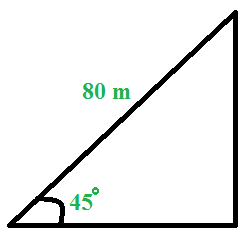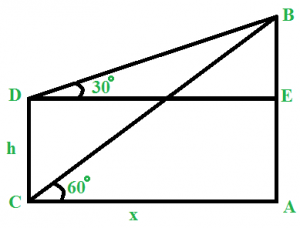# Trigonometry & Height and Distances

• Difficulty Level : Easy
• Last Updated : 07 Feb, 2019

From this topic, usually, questions from heights and distances is asked. Sometimes, it has been observed that straight forward questions from trigonometric ratios such as questions based on quadrants, small simplification questions, etc. have also been asked in addition to heights and distances. Concepts of trigonometry are applicable if and only if the triangle is a right angled triangle.

• π radian = 180 degrees
• sin θ = Perpendicular / Hypotenuse
cos θ = Base / Hypotenuse
tan θ = Perpendicular / Base
• In the first quadrant, all trigonometric ratios (sin, cos, tan, cosec, sec, cot) are positive.
In second quadrant, only sin and cosec are positive.
In third quadrant, only tan and cot are positive.
In fourth quadrant, only cos and sec are positive.
• sin2θ + cos2θ = 1
1 + tan2θ = sec2θ
1 + cot2θ = cosec2θ
• sin(- θ) = – sin θ
cos(- θ) = cos θ
tan(- θ) = – tan θ
cosec(- θ) = – cosec θ
sec(- θ) = sec θ
cot(- θ) = – cot θ
• ### Heights and Distances

Problems on height and distances are simply word problems that use trigonometry.

### Sample Problems

Question 1 : Simplify : [ (cos 80) / (sin 10) ] + cos 59 cosec 31
Solution : [ (cos 80) / (sin 10) ] + cos 59 cosec 31 = [ (cos (90 – 10)) / (sin 10) ] + cos 59 cosec (90 – 59)
=> [ (cos 80) / (sin 10) ] + cos 59 cosec 31 = (sin 10 / sin 10) + cos 59 sec 59
=> [ (cos 80) / (sin 10) ] + cos 59 cosec 31 = (sin 10 / sin 10) + cos 59 (1 / cos 59)
=> (sin 10 / sin 10) + cos 59 sec 59 = 1 + 1 = 2

Question 2 : From the top of alight house, the angles of depression of two ships are 30 and 45 degrees. The two ships, as it was observed from the top of the light house, were 100 m apart. Find the height of the light house.
Solution : here, we can apply the formula Height = Distance / [cot(original angle) – cot(final angle)]
=> Height of the light house = 100 / (cot 30 – cot 45) = 100 / (– 1) = 50+ 50 m

Question 3 : A 80 m long ladder is leaning on a wall. If the ladder makes an angle of 45 degrees with the ground, find the distance of the ladder from the wall.
Solution :Here, cos θ = Base / Hypotenuse
=> cos 45 = Base / 80
=> Base = 80 cos 45 = 80 /= 40=> Distance of the ladder from the wall = 40m

Question 4 : There are two poles, one on each side of the road. The higher pole is 54 m high. From the top of this pole, the angle of depression of top and bottom of the shorter pole is 30 and 60 degree respectively. Find the height of the shorter pole.
Solution :Let AB and CD be the two poles.
Let AC = x m and CD = h m
Now, in triangle ABC,
tan 60 = AB / AC
=>= 54 / AC
=> AC = 18m
Clearly, AC = DE = 18m
In triangle BED,
tan 30 = BE / DE
=> BE = DE tan 30
=> BE = 18/m
=> BE = 18 m
=> CD = AE = AB – BE
=> CD = 54 – 18 = 36 m
Therefore, height of the shorter pole = 36 m

### Problems on Trigonometry & Height and Distances | Set-2

This article has been contributed by Nishant Arora

Please write comments if you have any doubts related to the topic discussed above, or if you are facing difficulty in any question or if you would like to discuss a question other than those mentioned above.

Please write comments if you find anything incorrect, or you want to share more information about the topic discussed above

My Personal Notes arrow_drop_up
Recommended Articles
Page :